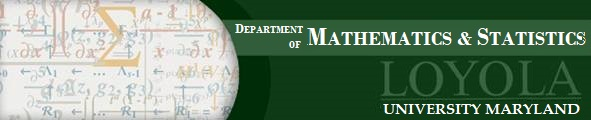## Dr. Michael Knapp

### Room: 175 Donnelly Science Hall

Abstract:

In this talk, we will study homogeneous additive polynomials in many variables. That is, we will consider polynomials of the form $$a_1 x_1^k +\cdots + a_n x_n^k$$ with integer coefficients. The Davenport-Lewis function $$\Gamma^*(k)$$ gives the smallest number n of variables which guarantees that this polynomial has a nontrivial zero in the ring $$\mathbb{Z}_p$$ of $$p$$-adic integers for every prime $$p$$. I will discuss two problems related to this function. First, I will briefly describe how to calculate the value of $$\Gamma^*(k)$$ for a specific number $$k$$. After that, I will discuss an old conjecture of Norton which says that we should have $$\Gamma^* (k) \equiv 1 \pmod k$$ for every integer $$k$$. I will prove that there are infinitely many counterexamples to this conjecture. The results I will present in the first part of the talk are joint work with former Loyola students Christopher Broll and Jessica Jennings, as well as other colleagues. The results in the second part are joint work with Hemar Godinho.

This talk will be accessible to Juniors and Seniors.

Seminar Flyer

Back to Current Seminars page